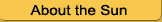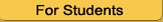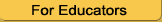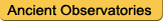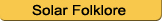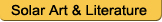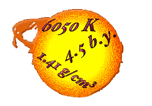Solar Math ProblemsDeveloped by Dr. Sten Odenwald, NASA.Summary of Activity: Students will learn mathematics and how they apply to the Sun, solar energy, space weather, and other space phenomena. The problems in this activity are designed for students in grades 3 through 12. This activity contains Sun-related problems from the NASA Space Math Website, where you can find many other astronomy-related math problems.Revised May 2010

Numbers and Operations

Problem 1 - Fractions in Space
Students explore the many ways that simple fractions come up in the study of planetary motion.
Grade: 3 - 5
Topics: working with fractions; time calculations.

Problem 2 - A Space Science Crossword Puzzle
Students work with positive and negative numbers to solve a crossword puzzle. Good exercise for pre-algebra review of adding and subtracting positive and negative numbers.
Grade: 4 - 6
Topics: Integer arithmetic; associative and distributive laws; positive and negative numbers.

Problem 3 - The Life Cycle of an Aurora
Students examine two eye-witness descriptions of an aurora and identify the common elements so that they can extract a common pattern of changes.
Grade: 4 - 6
Topics: Creating a timeline from narrative; ordering events by date and time.

Problem 4 - Solar Activity and Satellite Mathematics
When solar storms cause satellite problems, they can also cause satellites to lose money. The biggest source of revenue from communications satellites comes from transponders that relay television programs, ATM transactions and many other vital forms of information. They are rented to many different customers and can cost nearly \$2 million a year for each transponder. This activity examines what happens to a single satellite when space weather turns bad!
Grade: 4 - 6
Topics: Decimals; money; percents.

Problem 5 - Scientific Notation I
Scientists use scientific notation to represent very big and very small numbers. In this exercise, students will convert some 'astronomical' numbers into SN form.
Grade: 5 - 9
Topics: Scientific notation - conversion from decimal to SN.

Problem 6 - Scientific Notation II
In this continuation of the review of Scientific Notation, students will perform simple addition and subtraction problems
Grade: 5 - 9
Topics: Scientific notation - addition and subtraction.

Problem 7 - Scientific Notation III
In this continuation of the review of Scientific Notation, students will perform simple multiplication and division problems with an astronomy and space science focus.
Grade: 5 - 9
Topics: Scientific notation - multiplication and division.

Problem 8 - Data Corruption by High-Energy Particles
Students will see how solar flares can corrupt satellite data, and create a timeline for a spectacular episode of data loss recorded by the SOHO satellite using images obtained by the satellite. Students will also calculate the speed of the event as particles are ejected from the Sun and streak towards Earth.
Grade: 6 - 8
Topics: Time and speed calculations; interpreting scientific data.

Problem 9 - Working with rates.
Students examine mixed rates for a variety of situations and their connections to ratios.
Grade: 6 - 8
Topics:Ratios; scientific notation; unit conversion.

Problem 10 - The STEREO Mission: getting the message across
Students learn about how the transmission of data is affected by how far away a satellite is for the two satellites in the STEREO constellation.
Grade: 6 - 8
Topics:multiplication; division; decimal numbers.

Problem 11 - The November 8, 2004 solar storm.
Students calculate the speed of a CME, and describe their aurora observations through writing and drawing.
Grade: 6 - 8
Topics:Time calculations; distance = speed x time.

Problem 12 - How to make faint things stand out in a bright world!
Students learn that adding images together often enhances faint things not seen in only one image; the power of averaging data.
Grade: 6 - 8
Topics:Multiplication; division; decimal numbers.

Problem 13 - Unit Conversions
Students work with unit conversions and use them to solve a series of practical problems in science and solar energy.
Grade: 6 - 10
Topics: Unit Conversions.

Problem 14 - Unit Conversions II
Students work with more unit conversions that are a bit tricky.
Grade: 6 - 10
Topics: Unit Conversions.

Problem 15 - An Interplanetary Shock Wave
On November 8, 2000 the Sun released a coronal mass ejection that traveled to Earth, and its effects were detected on Jupiter and Saturn several weeks later. In this problem, students will use data from this storm to track its speed and acceleration as it traveled across the solar system.
Grade: 6 - 10
Topics:Time calculations; distance = speed x time.

Problem 16 - Solar Storm Timeline.
How long does a solar storm last? How fast does it travel? Students will examine an event timeline for a space weather event and use time addition and subtraction skills to calculate storm durations and speeds.
Grade: 7 - 9
Topics:Time math; decimal math; speed = distance/time.

Problem 17 - Scientific Notation - An Astronomical Perspective.
This collection of problems will have students reviewing how to perform multiplication and division with large and small numbers, while learning about some interesting astronomical applications. They will learn about the planet Osiris, how long it takes to download all of NASA's data archive, the time lag for radio signals to Pluto, and many more real-world applications.
Grade: 8 - 10
Topics:Scientific notation; decimal math.

Problem 18 - Ice or Water?
Whether a planetary surface contains ice or liquid water depends on how much heat is available. Students explore the concepts of Specific heat and Latent Heat of Fusion to better understand the and quantify the energy required for liquid water to exist under various conditions.
Grade: 8 - 10
Topics:Scientific notation; unit conversion.

Problem 19 - Water on Planetary Surfaces
Students work with watts and Joules to study melting ice.
Grade: 8 - 10
Topics:Unit conversion, rates.

Problem 20 - Solar Dynamics Observatory: Working with Giga, Tera, Peta and Exabytes.
The recent launch of SDO will bring 'high definition TV' to the study of the sun's surface details. This also means a HUGE amount of data will have to be processed every day to handle the torrent of information. This activity works with the prefixes giga, tera ,peta and exa to familiarize students with how to interpret these quantities in a practical settion. Students already know about 'gigabytes', but the SDO data stream represents terabytes per day, and petabytes per year in data storage demands.
Grade: 8 - 12
Topics:Powers of ten; time conversion: seconds, minutes, days, years.

Algebra and calculus

Problem 1 - A Bird's-Eye Look at the Sun-Earth System
Students solve simple equations for x, (like 2x + 3 = 5) to discover which words complete an essay on the causes of aurora, and answer questions after reading the completed essay.
Grade: 5 - 7
Topics: Solving for X; distributive law; associative law.

Problem 2 - Variables and Expressions from Around the Cosmos
Students evaluate linear equations describing a variety of astronomical situations.
Grade: 6 - 8
Topics: Evaluating simple one-variable equations.

Problem 3 - Equations with One Variable - Part I
Students solve formulas of the form 2001 = 1858 + 11x to find 'X'.
Topics: Topics: addition, subtraction, multiplication, division; solving simple equations.

Problem 4 - Equations with One Variable - Part II
Students work with equations like '4.3 = 3.26D' to solve for D in a number of simple astronomical problems involving distances, speed and temperature conversion. Grade: 6 - 8
Topics:Equations in one variable; multiplication; division; decimals.

Problem 5 - Can You Hear me now?
Students learn about how the transmission of data is affected by how far away a satellite is, for a variety of spacecraft in the solar system.
Grade: 6 - 8
Topics:Multiplication; division; decimal numbers.

Problem 6 - Magnetic Forces and Kinetic Energy
Students use the formula for the Kinetic Energy of a charged particle to calculate particle speeds for different voltages, and answer simple questions about lightning, aurora and Earth's radiation belts.
Grade: 6 - 8
Topics:Square root; time=speed x distance; decimal math; significant figures.

Problem 7 - Super-fast solar flares!
Students will analyze consecutive images taken of an erupting solar flare, and use the information provided to calculate the speed of the flare.
Grade: 6 - 9
Topics:Image scales; time calculations; speed calculations.

Problem 8 - Rates and Slopes: An astronomical perspective.
Students determine the slopes for two linear graphs and make the connection to rates with mixed units.
Grade: 7 - 9
Topics:Finding the slope of a linear graph.

Problem 9 - The Last Total Solar Eclipse-Ever!
Students explore the geometry required for a total solar eclipse, and estimate how many years into the future the last total solar eclipse will occur as the moon slowly recedes from Earth by 3 centimeters/year.
Grade: 7 - 10
Topics:Simple linear equations.

Problem 10 - The Comet Encke Tail Disruption Event
On April 20, 2007 NASA's STEREO satellite captured a rare impact between a comet and the fast-moving gas in a solar coronal mass ejection. In this problem, students analyze a STEREO satellite image to determine the speed of the tail disruption event.
Grade: 8 - 10
Topics:Time calculation; finding image scale; calculating speed from distance and time.

Problem 11 - Stellar Temperature, Size and Power
Students work with a basic equation to explore the relationship between temperature, surface area and power for a selection of stars.
Grade: 8 - 11
Topics:Algebra; derivatives.

Problem 12 - The Heliopause...a question of balance
Students will learn about the concept of pressure equilibrium by studying a simple mathematical model for the Sun's heliopause located beyond the orbit of Pluto. They will calculate the distance to the heliopause by solving for 'R' and then using an Excel spreadsheet to examine how changes in solar wind density, speed and interstellar gas density relate to the values for R.
Grade: 8 - 10
Topics:Formulas with two variables; scientific notation; spreadsheet programming.

Problem 13 - Systems of Equations in Space Science
This problem has students solve two problems involving three equations in three unknowns to learn about solar flares, and communication satellite operating power.
Grade: 8 - 10
Topics:Decimals, solving systems of equations, matrix math, algebraic substitution.

Problem 14 - Magnetic Energy From B to V
Students will use formulas for the volume of a sphere and cylinder, and magnetic energy, to calculate the total magnetic energy of two important 'batteries' for space weather phenomena- solar prominences and the Earth's magnetotail. This requires scientific notation, a calculator, and experience with algebraic equations with integer powers of 2 and 3.
Grade: 8 - 10
Topics:Algebra I; volumes; decimal math; scientific notation.

Problem 15 - The Distance to Earth's Magnetopause
Students use an algebraic formula and some real data, to calculate the distance from Earth to the magnetopause, where solar wind and magnetosphere pressure are in balance.
Grade: 8 - 10
Topics:Evaluating a function with two variables; completing tabular entries.

Problem 16 - Seeing Solar Storms in STEREO - I
Students work out the details of stereoscopic vision using elementary properties of triangles and the Law of Cosines to determine the distance from earth of a solar storm cloud.
Grade: 8 - 10
Topics:Geometry, Law of Cosines, V = D/T

Problem 17 - Seeing Solar Storms in STEREO - II
Students explore the geometry of stereo viewing by studying a solar storm viewed from two satellites.
Grade: 10 - 12
Topics:Geometry; Trigonometry.

Problem 18 - Oscillating Spheres
Many astronomical bodies have a natural period of oscillation. In this problem, students will use a simple mathematical model to calculate the period of oscillation of a star, a planet, and a neutron star from the estimated densities of these bodies.
Grade: 9 - 11
Topics:Algebra; calculating with a formula.

Problem 19 - Solar Flare Reconstruction
Students will use data from a solar flare to reconstruct its maximum emission using graphical estimation (pre-algebra), power-law function fitting (Algebra 2), and will determine the area under the profile (Calculus).
Grade: 9 - 11
Topics:Plotting tabular data; fitting functions; integration.

Problem 20 - The Pressure of a Solar Storm
Students will examine three mathematical models for determining how much pressure a solar storm produces as it affects Earth's magnetic field. They will learn that magnetism produces pressure, and that this accounts for many of the details seen in solar storms.
Grade: 9 - 11
Topics:Substituting numbers into equations; filling out missing table entries; data interpretation; mathematical models.

Problem 21 - Monster Functions in Space Science
This problem has students employ a pair of complicated algebraic equations to evaluate the strength of the Sun's magnetic field near Earth's orbit. The equations are a model of the Sun's magnetic field in space based on actual research by a solar physicist. This introduces students to a real-world application of mathematical modeling, and extracting predictions from theoretical models that can be tested. Students are provided the values for the relevant variables, and through substitution, calculate the numerical values for two 'vector' components of the Sun's magnetic field near Earth's orbit.
Grade: 9 - 11
Topics:Decimals, scientific notation, significant figures.

Problem 22 - Parametric Functions and Substitution
The relationship between the strength of a solar storm and the resulting magnetic disturbance on Earth is given as a series of equations. Students are asked to create new formulae based on these parametric these equations using the method of substitution.
Grade: 10 - 11
Topics:Algebraic manipulation, integer exponents, scientific notation, significant figures and rounding.

Problem 23 - Differentiation
Students explore partial derivatives by calculating rates of change in simple equations taken from astrophysics.
Grade: 11 - 12
Topics:Differentiation; algebra.

Problem 24 - Finding Mass in the Cosmos
Students derive a simple formula, then use it to determine the masses of objects in the universe from the orbit periods and distances of their satellites.
Grade: 9 - 12
Topics: Scientific Notation; Algebra II; parametric equations.

Problem 25 - The Internal Density and Mass of the Sun
Students use a simple, spherically symmetric density profile to determine the mass of the Sun using integral calculus.
Grade: 11 - 12
Topics:Algebra II; Polynomials; integral calculus.

Geometry, Area and Volume

Problem 1 - Solar Eclipses and Satellite Power
Students will create a scaled drawing of the orbits of three satellites around Earth, and calculate how long each satellite will be in the shadow of Earth. They will be asked to figure out how to keep the satellites operating even without sunlight to power their solar panels.
Grade: 5 - 8
Topics: Geometry, decimal math.

Problem 2 - Solar Power and Satellite Design
Students perform simple surface area calculations to determine how much solar power a satellite can generate, compared to the satellite's needs.
Grade: 5 - 8
Topics: Area of irregular polygons.

Problem 3 - How high is an aurora?
Students use the properties of a triangle to determine how high up aurora are. They also learn about the parallax method for finding distances to remote objects.
Grade: 5 - 8
Topics: Geometry; measuring angles.

Problem 4 - Solar Surface Details
Students will analyze a picture of a sunspot to learn more about its size, and examine the sizes of various other features on the surface of the Sun that astronomers study.
Grade: 6 - 8
Topics: Finding the scale of an image; metric measurement; decimal math.

Problem 5 - Hinode Satellite Power
Students will study the design of the Hinode solar satellite and calculate how much power it can generate from its solar panels.
Grade: 6 - 8
Topics: Area of rectangle,area of cylinder, unit conversion.

Problem 6 - Solar Flares and Sunspot Sizes
Students compare sunspot sizes to the frequency of solar flares and discover that there is no hard and fast rule that relates sunspot size to its ability to produce very large flares.
Grade: 6 - 8
Topics: Interpreting tabular data; percentages; decimal math.

Problem 7 - How fast does the Sun spin?
Students will analyze consecutive images taken by the Hinode satellite to determine the Sun's speed of rotation, and the approximate length of its 'day'.
Grade: 6 - 9
Topics:Image scales; time calculations; speed calculations, unit conversions.

Problem 8 - Observing the Sun's rotation
Students use a 'Sunspotter' telescope to track sunspots during the week of November 7, 2004, and calculate the rotation period of the Sun.
Note: This was meant as a lab exercise, and is provided here just as an example of how to do this when sunspots can be observed. For more information on how to observe the Sun safely, read the Observing the Sun section.
Alternatively, you can do this exercise with archived solar images. One way to find them is to go to MDI Intensitygrams, and browse the GIF images by month and year. Either way, you will need to superimpose a solar coordinate grid on your images!
Grade: 6 - 8
Topics: Lab exercise using a 'Sunspotter' telescope to measure Sun's rotation.

Problem 9 - Hinode Sees Mysterious Solar Micro-flares
Students will analyze an image taken by the Hinode solar satellite to determine the scale of the image in kilometers per millimeter, then use this to determine the sizes of solar micro-flares. From the number of micro-flares that they count in the image, the area of the image in square kilometers, and the surface area of a spherical sun, they will calculate the total number of micro-flares on the solar surface.
Grade: 6 - 9
Topics: Image scales; area calculation; unit conversions.

Problem 10 - Satellite Surface Area
Students calculate the surface area of an octagonal cylinder and calculate the power it would yield from solar cells covering its surface.
Grade: 7 - 9
Topics: Surface areas; hexagon; decimal math.

Problem 11 - Angular Size and velocity
Students study a spectacular photo of the ISS passing across the face of the Sun, and work out the angular sizes and speeds of the transit to figure out how long the event took in order to photograph it.
Grade: 8 - 10
Topics: Geometry; measuring angles.

Problem 12 - An Application of the Parallax Effect
The STEREO mission views the Sun from two different locations in space. By combining this data, the parallax effect can be used to determine how far above the solar surface various active regions are located. Students use the Pythagorean Theorem, a bit of geometry, and some actual STEREO data to estimate the height of Active Region AR-978.
Grade: 8 - 10
Topics: Pythagorean Theorem; square-root; solving for variables.

Problem 13 - A Lunar Transit of the Sun from Space
One of the STEREO satellites observed the disk of the moon pass across the Sun. Students will use simple geometry to determine how far the satellite was from the moon and Earth at the time the photograph was taken.
Grade: 8 - 10
Topics: Geometry; parallax; arithmetic.

Problem 14 - Getting an Angle on the Sun and Moon
Students explore angular size and scale by comparing two images of the sun and moon which have identical angular size, but vastly different scales.
Grade: 8 - 10
Topics: Geometry; angle measure; area; proportion.

Problem 15 - The Transit of Mercury
As seen from Earth, the planet Mercury occasionally passes across the face of the Sun; an event that astronomers call a transit. From images taken by the Hinode satellite, students will create a model of the solar disk to the same scale as the image, and calculate the distance to the Sun.
Grade: 9 - 11
Topics: Image scales; angular measure; degrees, minutes and seconds.

Problem 16 - Loopy Sunspots!
Students will analyze data from the Hinode satellite to determine the volume and mass of a magnetic loop above a sunspot. From the calculated volume, based on the formula for the volume of a cylinder, they will use the density of the plasma determined by the Hinode satellite to determine the mass in tons of the magnetically trapped material.
Grade: 9 - 11
Topics: Image scales; cylinder volume calculation; scientific notation; unit conversions.

Problem 17 - Seeing Solar Storms in STEREO - I
Students work out the details of stereoscopic vision using elementary properties of triangles and the Law of Cosines to determine the distance from earth of a solar storm cloud.
Grade: 8 - 10
Topics:Geometry, Law of Cosines, V = D/T

Problem 18 - Seeing Solar Storms in STEREO - II
Students explore the geometry of stereo viewing by studying a solar storm viewed from two satellites.
Grade: 10 - 12
Topics:Geometry; Trigonometry.

Measurement, scale, and speed calculation

Problem 1 - The relative sizes of the Sun and stars
Students work through a series of comparisons of the relative size of the Sun compared to other stars, to create a scale model of stellar sizes using simple fractional relationships. ( e.g if Star A is 6 times larger than Star B, and Star C is 1/2 the size of Star B, how big is Star C in terms of Star A?)
Grade: 4 - 6
Topics: Working with fractions; scale models.

Problem 2 - The Auroral Oval
Students learn that the aurora are observed as two 'halos' of light encircling the North and South Poles. Students use measurements made from two satellite images of the 'auroral ovals' to determine the diameter of the rings, and their approximate geographic centers - which are not at the geographic poles!
Grade: 5 - 7
Topics: Finding the scale of an image; measurement; decimal math.

Problem 3 - Measuring the Speed of a Solar Tsunami!
Recent data from the Hinode satellite is used to measure the speed of a solar explosion on the surface of the Sun using a series of images taken by the satellite at three different times. Students calculate the speed of the blast between the first pair and last pair of images, and determine if the blast wave was accelerating or decelerating in time.
Grade: 5 - 8
Topics: Finding image scale; calculating time differences; calculating speed from distance and time.

Problem 4 - Monster Sunspots!
Some sunspots are so big that they can be seen from Earth without a telescope. In this problem, students will use images of three super-spots and calculate their sizes from the image scaling information. They will then order the images from the smallest super-spot to the largest super-spot.
Grade: 5 - 9
Topics: Multiplication; calculating length from image scale.

Problem 5 - Hinode: Close-up of a Sunspot
Students will determine the sizes of sunspots and solar granulation cells from a recent image taken by the Hinode solar observatory.
Grade: 6 - 8
Topics: Image scales, metric units, unit conversion.

Problem 6 - The Hinode satellite views the Sun
Students will use a full-Sun image from the new Hinode satellite to sketch the locations of magnetic fields on the Sun's surface using information in the introductory article as a guide.
Grade: 6 - 8
Topics: Image interpretation; hand-eye coordination; reading to be informed.

Problem 7 - Moving Magnetic Filaments Near Sunspots
Students will use two images from the new, Hinode (Solar-B) solar observatory to calculate the speed of magnetic filaments near a sunspot. The images show the locations of magnetic features at two different times. Students calculate the image scales in kilometers/mm and determine the time difference to estimate the speeds of the selected features.
Grade: 6 - 8
Topics: scaling, estimation, speed calculations, time arithmetic.

Problem 8 - Measuring Speed in the Universe.
In this activity, students measure the speed of astronomical phenomena using the scaling clues and the time intervals between photographs of three phenomena: A supernova explosion, a coronal mass ejection, and a solar flare shock wave.
Topics: Measuring, scaling, speed calculations.

Problem 9 - SDO: Measuring the Speed of an Eruptive Prominence.
Students use recent First Light images of the Sun from SDO to calculate the speed of a prominence using a sequence of scaled images and computing position shift over the time interval of the images.
Topics: Measuring, scaling, speed calculations.

Problem 10 - SDO Reveals Details on the Surface of the Sun.
Students use a spectacular colored image of the Sun to calculate the scale of the image in kilometers per millimeter, and then search for the smallest features relative to the size of Earth.
Topics: Measurement, scale, proportion.

Problem 11 - Changing Perspectives on the Sun's Diameter.
Students compare two images of the Sun taken by the SOHO satellite to measure the apparent diameter change from different Earth orbit locations in the winter and summer.
Topics: Measurement; parallax; metric units; percentage change.

Problem 12 - STEREO Watches the Sun Kick Up a Storm.
Students use images from the STEREO observation of a 'solar tsunami' to estimate its speed and kinetic energy.
Topics: metric measurement; scaling; speed calculation; evaluating a simple energy equation.

Data Analysis and Probability

Problem 1 - Cosmic Bar Graphs
Students interpret simple bar graphs taken from astronomical data.
Grade: 3 - 5
Topics: Finding maxima and minima; fractions; extrapolating data.

Problem 2 - Solar Storms: Sequences and Probabilities - Part I
Students work out the probabilities for various combinations of solar storms during a given week.
Grade: 4 - 7
Topics: Probability; numerating possible outcomes.

Problem 3 - Solar Storms: Sequences and Probabilities - Part II
Students continue their study of a stormy week on the Sun by working out the probabilities for joint events.
Grade: 4 - 7
Topics: Probability; numerating possible outcomes.

Problem 4 - Solar Storms - Fractions and Percentages
Students create a Venn Diagram to summarize data on a series of solar storms, and determine how often solar flares occur when a solar plasma eruption happens.
Grade: 4 - 7
Topics:Precentages; fractions; Venn Diagramming.

Problem 5 - Aurora Power!
Students use data to estimate the power of an aurora, and compare it to common things such as the electrical consumption of a house, a city and a country.
Grade: 5 - 7
Topics: Interpreting tabulated data.

Problem 6 - Solar Flares, CME's and Aurora
Some articles about the Northern Lights imply that solar flares cause them. Students will use data to construct a simple Venn Diagram, and answer an important question about whether solar flares cause CME's and Aurora.
Grade: 5 - 7
Topics: Venn Diagramming.

Problem 7 - The Space Station Orbit Decay and Space Weather
Students will learn about the continued decay of the orbit of the International Space Station by studying a graph of the Station's altitude versus time. They will calculate the orbit decay rates, and investigate why this might be happening.
Grade: 5 - 8
Topics: Interpreting graphical data; decimal math.

Problem 8 - Do Fast CMEs Produce SPEs?
Recent data on solar proton storms (SPEs) and coronal mass ejections (CMEs) are compared using Venn Diagrams to see if the speed of a CME makes solar proton storms more likely or not.
Grade: 5 - 8
Topics: Venn Diagrams; counting; calculating percentages and odds.

Problem 9 - Solar Storm Energy and Pie Graphs
Students study two Pie graphs describing solar flares and draw conclusions about percentages and their various forms of energy.
Grade: 6 - 8
Topics: Interpreting Pie Charts.

Problem 10 - Solar Storms: Odds, Fractions and Percentages
Students will use actual data on solar storms to learn about the different kinds of storms and how common they are. This is a basic science activity that professionals do in order to look for relationships between different kinds of events that might lead to clues about what causes them. Can your students come up with something new that no one has thought about before? The Venn Diagramming activity is a key element of the activity and is reasonably challenging!
Grade: 6 - 8
Topics: Averaging; fractions; percentages; odds; Arithmetic Operations; Venn Diagrams.

Problem 11 - A Magnetic Case for 'What Came First?
Students create a timeline for events based on several data plots from the THEMIS program, and use their timeline to answer questions about the causes of magnetic storms.
Grade: 6 - 8
Topics: Time calculations.

Problem 12 - Satellite Failures and the Sunspot Cycle
There are over 1500 working satellites orbiting Earth, representing an investment of 160 billion dollars. Every year, between 10 and 30 of these re-enter the atmosphere. In this problem, students compare the sunspot cycle with the record of satellites re-entering the atmosphere and determine if there is a correlation. They also investigate how pervasive satellite technology has become in their daily lives.
Grade: 6 - 8
Topics: Graphing tabular data; decimal math.

Problem 13 - The Sunspot Cycle - endings and beginnings
Students will examine a plot of the sunspot cycle and extract information from the plotted data about the previous sunspot cycle, and make predictions about the next one about to start in 2007.
Grade: 6 - 9
Topics: Graph reading; extrapolation; time calculations.

Problem 14 - Super-sized Sunspots and the Solar Cycle.
Students compare the dates of the largest sunspots since 1900 with the year of the peak sunspot cycle. They check to see if superspots are more common after sunspot maximum or before. They also compare superspot sizes with the area of Earth.
Grade: 6 - 8
Topics: Interpreting tabular data; decimal math

Problem 15 - Magnetic Storms I
Students learn about magnetic storms using real data in the form of a line graph. They answer simple questions about data range, maximum, and minimum.
Grade: 7 - 9
Topics: Interpreting a graph; time calculations.

Problem 16 - Magnetic Storms II
Students learn about the Kp index using a bar graph. They use the graph to answer simple questions about maxima and time.
Grade: 7 - 9
Topics: Interpreting a graph; time calculations.

Problem 17 - Solar Proton Events and Satellite Damage
Students will examine the statistics for Solar Proton Events since 1996 and estimate their damage to satellite solar power systems.
Grade: 7 - 9
Topics: Interpreting tabular data; histogramming.

Problem 18 - Solar Insolation Changes and the Sunspot Cycle
Students compare changes in the amount of solar energy reaching earth with the 11-year sunspot cycle to predict the impact on designing a photovoltaic system for a home.
Grade: 8 - 10
Topics: Graph analysis, correlations, kilowatt, kilowatt-hours.

Problem Solving

Problem 1 - Solar Activity and Tree Rings - What's the connection?
Trees require sunlight to grow, and we know that solar activity varies with the sunspot cycle. Can an average tree sense solar activity cycles and change the way it grows from year to year? This activity uses a single tree to compare its growth rings to the sunspot cycle. This is also an interesting suggestion for science fair projects!
Here is the Completed Excel Spreadsheet for the teacher's guide.
Grade: 4 - 6
Topics: Spreadsheets and technology; decimal math.

Problem 2 - Solar Storm Timeline
Students read a narrative about the events involved in a solar storm, creates a chronology for the sequence of events, and answer some simple time-related questions.
Grade: 6 - 8
Topics: Time calculations.

Problem 3 - Airline Travel and Space Weather
Students will read an excerpt from the space weather book 'The 23rd Cycle' by Dr. Sten Odenwald, and answer questions about airline travel during solar storms. They will learn about the natural background radiation they are exposed to every day, and compare this to radiation dosages during jet travel.
Grade: 6 - 8
Topics: Reading to be informed; decimal math.

Problem 4 - Solar Storms in the News
Students will use a newspaper archive to explore how reporters have described the causes of aurora since the 1850's. They will see how some explanations were popular for a time, then faded into oblivion, as better scientific explanations were created.
Grade: 6 - 10
Topics: Online research; tallying data.

Problem 5 - Solar Energy in Space
Students will calculate the area of a satellite's surface being used for solar cells from an actual photo of the IMAGE satellite. They will calculate the electrical power provided by this one panel. Students will have to calculate the area of an irregular region using nested rectangles.
Grade: 7 - 10
Topics: Area of an irregular polygon; decimal math.

Problem 6 - A Mathematical Model of the Sun
Students will use the formula for a sphere and a shell to calculate the mass of the Sun for various choices of its density. The goal is to reproduce the measured mass and radius of the Sun by a careful selection of its density in a core region and a shell region. Students will manipulate the values for density and shell size to achieve the correct total mass. This can be done by hand, or by programming an Excel spreadsheet.
Grade: 8 - 10
Topics: Scientific notation; volume of a sphere and a spherical shell; density, mass and volume.

Problem 7 - CME Kinetic Energy and Mass
Coronal Mass Ejections (CMEs) are giant clouds of plasma released by the Sun at millions of kilometers per hour. In this activity, students calculate the kinetic energy and mass of several CMEs to determine typical mass ranges and speeds. Students will use the formula for kinetic energy to fill-in the missing entries in a table. They will then use the completed table to answer some basic questions about CMEs.
Grade: 8 - 10
Topics: Time calculation; evaluating a simple equation; solving for variables.

 Let Us Know: How did this lesson work in your classroom?

 Home · Request Solar Posters · Site Map · Glossary · About Us · Contact Us ©2010 by Stanford SOLAR Center · Permitted Uses · Credits